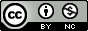Produced with R version 3.6.1 and pomp version 2.2.

## Objectives

Students completing this lesson will:

1. Gain an understanding of the nature of the problem of likelihood computation for POMP models.
2. Be able to explain the simplest particle filter algorithm.
3. Gain experience in the visualization and exploration of likelihood surfaces.
4. Be able to explain the tools of likelihood-based statistical inference that become available given numerical accessibility of the likelihood function.

## Overview

• The following schematic diagram represents conceptual links between different components of the methodological approach we’re developing for statistical inference on epidemiological dynamics.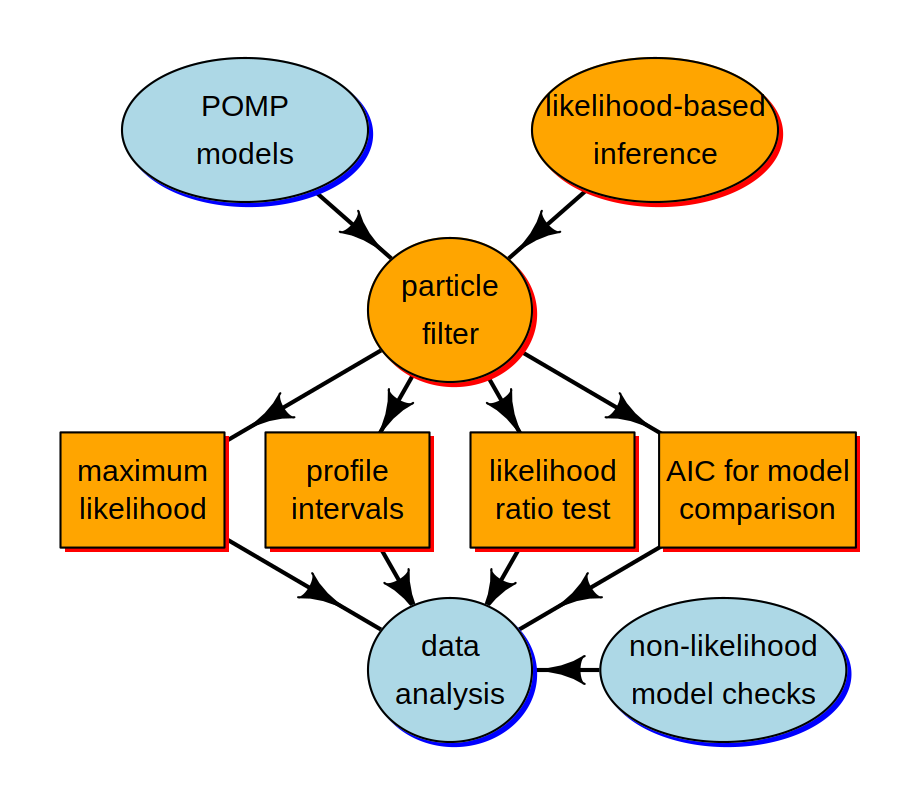• In this lesson, we’re going to discuss the orange compartments.

• The Monte Carlo technique called the particle filter is central for connecting the higher-level ideas of POMP models and likelihood-based inference to the lower-level tasks involved in carrying out data analysis.

• We employ a standard toolkit for likelihood based inference: Maximum likelihood estimation, profile likelihood confidence intervals, likelihood ratio tests for model selection, and other likelihood-based model comparison tools such as AIC.

• We seek to better understand these tools, and to figure out how to implement and interpret them in the specific context of POMP models.

## The likelihood function

• The basis for modern frequentist, Bayesian, and information-theoretic inference.

• Method of maximum likelihood introduced by Fisher (1922).

• The likelihood function itself is a representation of the what the data have to say about the parameters.

• A good general reference on likelihood is by Pawitan (2001).

### Definition of the likelihood function

• Data are a sequence of $$N$$ observations, denoted $$y_{1:N}^*$$.

• A statistical model is a density function $$f_{Y_{1:N}}(y_{1:N};\theta)$$ which defines a probability distribution for each value of a parameter vector $$\theta$$.

• To perform statistical inference, we must decide, among other things, for which (if any) values of $$\theta$$ it is reasonable to model $$y^*_{1:N}$$ as a random draw from $$f_{Y_{1:N}}(y_{1:N};\theta)$$.

• The likelihood function is $\mathcal{L}(\theta) = f_{Y_{1:N}}(y^*_{1:N};\theta),$ the density function evaluated at the data.

• It is often convenient to work with the log likelihood function, $\ell(\theta)= \log \mathcal{L}(\theta) = \log f_{Y_{1:N}}(y^*_{1:N};\theta).$

### Modeling using discrete and continuous distributions

• Recall that the probability distribution $$f_{Y_{1:N}}(y_{1:N};\theta)$$ defines a random variable $$Y_{1:N}$$ for which probabilities can be computed as integrals of $$f_{Y_{1:N}}(y_{1:N};\theta)$$.

• Specifically, for any event $$E$$ describing a set of possible outcomes of $$Y_{1:N}$$, $\mathbb{P}\left[{Y_{1:N} \in E}\right] = \int_E f_{Y_{1:N}}(y_{1:N};\theta)\, dy_{1:N}.$

• If the model corresponds to a discrete distribution, then the integral is replaced by a sum and the probability density function is called a probability mass function.

• The definition of the likelihood function remains unchanged. We will use the notation of continuous random variables, but all the methods apply also to discrete models.

## Indirect specification of a statistical model via a simulation procedure

• For simple statistical models, we may describe the model by explicitly writing the density function $$f_{Y_{1:N}}(y_{1:N};\theta)$$. One may then ask how to simulate a random variable $$Y_{1:N}\sim f_{Y_{1:N}}(y_{1:N};\theta)$$.

• For many dynamic models it is much more convenient to define the model via a procedure to simulate the random variable $$Y_{1:N}$$. This implicitly defines the corresponding density $$f_{Y_{1:N}}(y_{1:N};\theta)$$.

• For a complicated simulation procedure, it may be difficult or impossible to write down or even compute $$f_{Y_{1:N}}(y_{1:N};\theta)$$ exactly.

• It is important to bear in mind that the likelihood function exists even when we don’t know what it is! We can still talk about the likelihood function, and develop numerical methods that take advantage of its statistical properties.

## The likelihood for a POMP model

• Recall the following schematic diagram, showing dependence among variables in a POMP model.
• Measurements, $$Y_n$$, at time $$t_n$$ depend on the latent process, $$X_n$$, at that time.
• The Markov property asserts that latent process variables depend on their value at the previous timestep.
• To be more precise, the distribution of the state $$X_{n+1}$$, conditional on $$X_{n}$$, is independent of the values of $$X_{k}$$, $$k<n$$ and $$Y_{k}$$, $$k\le n$$.
• Moreover, the distribution of the measurement $$Y_{n}$$, conditional on $$X_{n}$$, is independent of all other variables.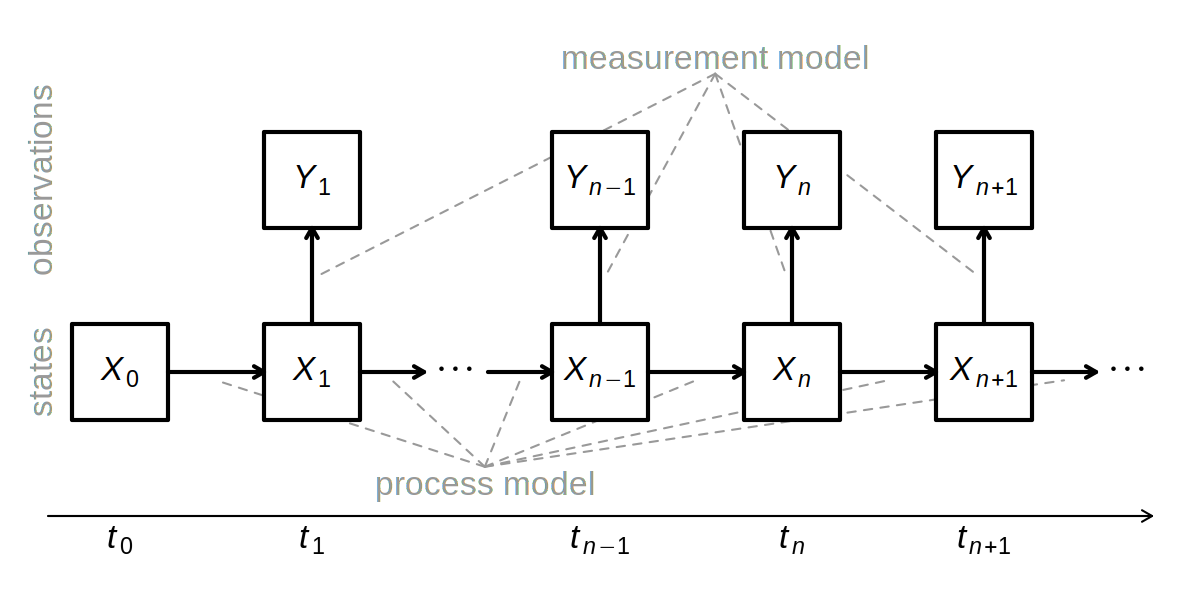• The latent process $$X(t)$$ may be defined at all times, but we are particulary interested in its value at observation times. Therefore, we write $X_n=X(t_n).$

• We write collections of random variables using the notation $$X_{0:N}=(X_0,\dots,X_N)$$.

• The one-step transition density, $$f_{X_n|X_{n-1}}(x_n|x_{n-1};\theta)$$, together with the measurement density, $$f_{Y_n|X_n}(y_n|x_n;\theta)$$ and the initial density, $$f_{X_0}(x_0;\theta)$$, specify the entire joint density via

$\begin{eqnarray} &&f_{X_{0:N},Y_{1:N}}(x_{0:N},y_{1:N};\theta)\\ && \quad = f_{X_0}(x_0;\theta)\,\prod_{n=1}^N\!f_{X_n | X_{n-1}}(x_n|x_{n-1};\theta)\,f_{Y_n|X_n}(y_n|x_n;\theta). \end{eqnarray}$

• The marginal density for sequence of measurements, $$Y_{1:N}$$, evaluated at the data, $$y_{1:N}^*$$, is $\mathcal{L}(\theta) = f_{Y_{1:N}}(y^*_{1:N};\theta)=\int\!f_{X_{0:N},Y_{1:N}}(x_{0:N},y^*_{1:N};\theta)\, dx_{0:N}.$

#### Special case: deterministic latent process

• When the latent process is non-random, the log likelihood for a POMP model closely resembles a nonlinear regression model.

• In this case, we can write $$X_{n}=x_n(\theta)$$, and the log likelihood is $\ell(\theta)= \sum_{n=1}^N \log f_{Y_n|X_n}\big(y_n^*| x_n(\theta); \theta\big).$

• If we have a Gaussian measurement model, where $$Y_n$$ given $$X_n=x_n(\theta)$$ is conditionally normal with mean $$\hat{y}_n\big(x_n(\theta)\big)$$ and constant variance $$\sigma^2$$, then the log likelihood contains a sum of squares which is exactly the criterion that nonlinear least squares regression seeks to minimize.

• More details on deterministic latent process models are given as a supplement.

#### General case: stochastic unobserved state process

• For a POMP model, the likelihood takes the form of an integral:

$\begin{eqnarray} \mathcal{L}(\theta) &=& f_{Y_{1:N}}({y^*_{1:N}};\theta) \\ &=& \! \int_{x_{0:N}} \!\! f_{X_0}(x_0;\theta)\prod_{n=1}^{N}\!f_{Y_n|X_n}({y^*_n}| x_n; \theta)\, f_{X_n|X_{n-1}}(x_n|x_{n-1};\theta)\, dx_{0:N}. \tag{L1} \end{eqnarray}$

• This integral is high dimensional and, except for the simplest cases, can not be reduced analytically.

## Monte Carlo likelihood by direct simulation

• We work toward introducing the particle filter by first proposing a simpler method that usually doesn’t work on anything but very short time series.

• Although this section is a demonstration of what not to do, it serves as an introduction to the general approach of Monte Carlo integration.

• First, let’s rewrite the likelihood integral using an equivalent factorization. As an exercise, you could check how the equivalence of Eqn. L1 and Eqn. L2 follows algebraically from the Markov property and the definition of conditional density.

$\begin{eqnarray} \mathcal{L}(\theta) &=& f_{Y_{1:N}}({y^*_{1:N}};\theta) \\ &=& \! \int_{x_{0:N}} \left\{ \prod_{n=1}^{N}\!f_{Y_n|X_n}({y^*_n}| x_n; \theta)\right\} f_{X_{0:N}}(x_{0:N};\theta)\, dx_{0:N}. \tag{L2} \end{eqnarray}$

• Notice, using the representation in Eqn. L2, that the likelihood can be written as an expectation, $\mathcal{L}(\theta) = \mathbb{E}\left[{\prod_{n=1}^{N}\!f_{Y_n|X_n}({y^*_n}| X_n; \theta)}\right],$ where the expectation is taken with $$X_{0:N}\sim f_{X_{0:N}}(x_{0:N};\theta)$$.

• Now, using a law of large numbers, we can approximate an expectation by the average of a Monte Carlo sample. Thus, $\mathcal{L}(\theta) \approx \frac{1}{J} \sum_{j=1}^{J}\prod_{n=1}^{N}\!f_{Y_n|X_n}({y^*_n}| X^j_n; \theta),$ where $$\{X^j_{0:N}, j=1,\dots,J\}$$ is a Monte Carlo sample of size $$J$$ drawn from $$f_{X_{0:N}}(x_{0:N};\theta)$$.

• We see that, if we generate trajectories by simulation, all we have to do to get a Monte Carlo estimate of the likelihood is evaluate the measurement density of the data at each trajectory and average.

• We get the plug-and-play property that our algorithm depends on rprocess but does not require dprocess.

• However, this naive approach scales poorly with dimension. It requires a Monte Carlo effort that scales exponentially with the length of the time series, and so is infeasible on anything but a short data set.

• One way to see this is to notice that, once a simulated trajectory diverges from the data, it will seldom come back. Simulations that lose track of the data will make a negligible contribution to the likelihood estimate. When simulating a long time series, almost all the simulated trajectories will eventually lose track of the data.

• We can see this happening in practice for the measles outbreak data: supplementary material

## Sequential Monte Carlo: The particle filter

• Fortunately, we can compute the likelihood for a POMP model by a much more efficient algorithm than direct Monte Carlo integration.

• We proceed by factorizing the likelihood in a different way: $\begin{eqnarray} \mathcal{L}(\theta)&=&f_{Y_{1:N}}(y^*_{1:N}; \theta) \\ &=& \prod_{n=1}^N\,f_{Y_n|Y_{1:n-1}}(y^*_n|y^*_{1:n-1};\theta) \\ &=& \prod_{n=1}^N\,\int f_{Y_n|X_n}(y^*_n|x_n;\theta)\,f_{X_n|Y_{1:n-1}}(x_n|y^*_{1:n-1};\theta)\, dx_{n}, \end{eqnarray}$ with the understanding that $$f_{X_1|Y_{1:0}}=f_{X_1}$$.

• The Markov property leads to the prediction formula:

$\begin{eqnarray} &&f_{X_n|Y_{1:n-1}}(x_n|y^*_{1:n-1}; \theta) \\ &&\quad = \int_{x_{n-1}} \! f_{X_n|X_{n-1}}(x_n|x_{n-1};\theta)\, f_{X_{n-1}|Y_{1:n-1}}(x_{n-1}| y^*_{1:n-1}; \theta) \, dx_{n-1}. \end{eqnarray}$

• Bayes’ theorem gives the filtering formula:

$\begin{eqnarray} &&f_{X_n|Y_{1:n}}(x_n|y^*_{1:n}; \theta) \\ &&\quad = f_{X_n|Y_n,Y_{1:n-1}}(x_n|y^*_n,y^*_{1:n-1}; \theta) \\ &&\quad =\frac{f_{Y_n|X_n}(y^*_{n}|x_{n};\theta)\,f_{X_n|Y_{1:n-1}}(x_{n}|y^*_{1:n-1};\theta)}{\int f_{Y_n|X_n}(y^*_{n}|u_{n};\theta)\,f_{X_n|Y_{1:n-1}}(u_{n}|y^*_{1:n-1};\theta)\, du_n}. \end{eqnarray}$

• This suggests that we keep track of two key distributions at each time $$t_n$$,

• The prediction distribution is $$f_{X_n | Y_{1:n-1}}(x_n| y^*_{1:n-1})$$.

• The filtering distribution is $$f_{X_{n} | Y_{1:n}}(x_n| y^*_{1:n})$$.

• The prediction and filtering formulas give us a recursion:

• The prediction formula gives the prediction distribution at time $$t_n$$ using the filtering distribution at time $$t_{n-1}$$.
• The filtering formula gives the filtering distribution at time $$t_n$$ using the prediction distribution at time $$t_n$$.
• The particle filter use Monte Carlo techniques to sequentially estimate the integrals in the prediction and filtering recursions. Hence, the alternative name of sequential Monte Carlo (SMC).

• A basic particle filter is described as follows:

1. Suppose $$X_{n-1,j}^{F}$$, $$j=1,\dots,J$$ is a set of $$J$$ points drawn from the filtering distribution at time $$t_{n-1}$$.
2. We obtain a sample $$X_{n,j}^{P}$$ of points drawn from the prediction distribution at time $$t_n$$ by simply simulating the process model: $X_{n,j}^{P} \sim \mathrm{process}(X_{n-1,j}^{F},\theta), \qquad j=1,\dots,J.$
3. Having obtained $$x_{n,j}^{P}$$, we obtain a sample of points from the filtering distribution at time $$t_n$$ by resampling from $$\big\{X_{n,j}^{P},j\in 1:J\big\}$$ with weights $w_{n,j}=f_{Y_n|X_n}(y^*_{n}|X^P_{n,j};\theta).$
4. The Monte Carlo principle tells us that the conditional likelihood $\begin{eqnarray} \mathcal{L}_n(\theta) &=& f_{Y_n|Y_{1:n-1}}(y^*_n|y^*_{1:n-1};\theta)\\ &=& \int f_{Y_n|X_n}(y^*_{n}|x_{n};\theta)\,f_{X_n|Y_{1:n-1}}(x_{n}|y^*_{1:n-1};\theta)\, dx_n \end{eqnarray}$ is approximated by $\hat{\mathcal{L}}_n(\theta)\approx\frac{1}{J}\,\sum_j\,f_{Y_n|X_n}(y^*_{n}|X_{n,j}^{P};\theta)$ since $$X_{n,j}^{P}$$ is approximately a draw from $$f_{X_n|Y_{1:n-1}}(x_{n}|y^*_{1:n-1};\theta)$$.
5. We can iterate this procedure through the data, one step at a time, alternately simulating and resampling, until we reach $$n=N$$.
6. The full log likelihood then has approximation

\begin{aligned} \ell(\theta)&=\log{{\mathcal{L}}(\theta)}\\ &=\sum_n \log{{\mathcal{L}}_n(\theta)}\\ &\approx \sum_n\log\hat{\mathcal{L}}_n(\theta). \end{aligned}

• Key references on the particle filter include Kitagawa (1987), Arulampalam et al. (2002), and the book by Doucet et al. (2001). Pseudocode for the above is provided by King et al. (2016).

• It can be shown that the particle filter provides an unbiased estimate of the likelihood. This implies a consistent but biased estimate of the log likelihood.

## Particle filtering in pomp

Here, we’ll get some practical experience with the particle filter, and the likelihood function, in the context of our measles-outbreak case study. Here, we simply repeat the construction of the SIR model we looked at earlier.

In pomp, the basic particle filter is implemented in the command pfilter. We must choose the number of particles to use by setting the Np argument.

measSIR %>%
pfilter(Np=5000) -> pf
logLik(pf)
##  -273.2273

We can run a few particle filters to get an estimate of the Monte Carlo variability:

replicate(10,
measSIR %>% pfilter(Np=5000)
) -> pf
ll <- sapply(pf,logLik); ll
##   -267.6608 -273.9764 -362.0711 -292.3213 -281.3281 -273.2918 -242.8558
##   -238.5864 -220.1018 -275.2621
logmeanexp(ll,se=TRUE)
##                    se
## -222.40443   16.62362

## Review of likelihood-based inference

For now, suppose that software exists to evaluate and maximize the likelihood function, up to a tolerable numerical error, for the dynamic models of interest. Our immediate task is to think about how to use that capability.

• Likelihood-based inference (meaning statistical tools based on the likelihood function) provides tools for parameter estimation, standard errors, hypothesis tests and diagnosing model misspecification.

• Likelihood-based inference often (but not always) has favorable theoretical properties. Here, we are not especially concerned with the underlying theory of likelihood-based inference. On any practical problem, we can check the properties of a statistical procedure by simulation experiments.

### The maximum likelihood estimate (MLE)

• A maximum likelihood estimate (MLE) is $\hat\theta = \underset{\theta}{\arg\max} \ell(\theta),$ where $$\underset{\theta}{\arg\max} g(\theta)$$ means a value of argument $$\theta$$ at which the maximum of the function $$g$$ is attained, so $$g\big(\underset{\theta}{\arg\max} g(\theta)\big) = \max_\theta g(\theta)$$.

• If there are many values of $$\theta$$ giving the same maximum value of the likelihood, then an MLE still exists but is not unique.

• Note that $$\underset{\theta}{\arg\max} \mathcal{L}(\theta)$$ and $$\underset{\theta}{\arg\max} \ell(\theta)$$ are the same. Why?

### Standard errors for the MLE

• Of course, we have a responsibility to attach a measure of uncertainty to our parameter estimates!

• Usually, this means obtaining a confidence interval, or in practice an interval close to a true confidence interval which should formally be called an approximate confidence interval. In practice, the word “approximate” is often dropped!

• There are three main approaches to estimating the statistical uncertainty in an MLE.
1. The Fisher information.
• A computationally quick approach when one has access to satisfactory numerical second derivatives of the log likelihood.
• The approximation is satisfactory only when $$\hat\theta$$ is well approximated by a normal distribution.
• Neither of the two requirements above are typically met for POMP models.
• A review of standard errors via Fisher information is provided as a supplement.
2. Profile likelihood estimation. This approach is generally preferable to the Fisher information for POMP models.
3. A simulation study, also known as a bootstrap.
• If done carefully and well, this can be the best approach.
• A confidence interval is a claim about reproducibility. You claim, so far as your model is correct, that on 95% of realizations from the model, a 95% confidence interval you have constructed will cover the true value of the parameter.
• A simulation study can check this claim fairly directly, but requires the most effort.
• The simulation study takes time for you to develop and debug, time for you to explain, and time for the reader to understand and check what you have done. We usually carry out simulation studies to check our main conclusions only.
• Further discussion of bootstrap methods for POMP models is provided as a supplement.

### Confidence intervals via the profile likelihood

• Let’s consider the problem of obtaining a confidence interval for the first component of $$\theta$$. We’ll write $\theta=(\phi,\psi).$

• The profile log likelihood function of $$\phi$$ is defined to be $\ell_\mathrm{profile}(\phi) = \max_{\psi}\ell(\phi,\psi).$ In general, the profile likelihood of one parameter is constructed by maximizing the likelihood function over all other parameters.

• Note that, $$\max_{\phi}\ell_\mathrm{profile}(\phi) = \max_{\theta}\ell(\theta)$$ and that maximizing the profile likelihood $$\ell_\mathrm{profile}(\phi)$$ gives the MLE, $$\hat{\theta}$$. Why?

• An approximate 95% confidence interval for $$\phi$$ is given by $\big\{\phi : \ell(\hat\theta) - \ell_\mathrm{profile}(\phi) < 1.92\big\}.$

• This is known as a profile likelihood confidence interval. The cutoff $$1.92$$ is derived using Wilks’s theorem, which we will discuss in more detail when we develop likelihood ratio tests.

• Although the asymptotic justification of Wilks’s theorem is the same limit that justifies the Fisher information standard errors, profile likelihood confidence intervals tend to work better than Fisher information confidence intervals when $$N$$ is not so large—particularly when the log likelihood function is not close to quadratic near its maximum.

## The graph of the likelihood function: The likelihood surface

• It is extremely useful to visualize the geometric surface defined by the likelihood function.

• If $$\Theta$$ is two-dimensional, then the surface $$\ell(\theta)$$ has features like a landscape.

• Local maxima of $$\ell(\theta)$$ are peaks.

• Local minima are valleys.

• Peaks may be separated by a valley or may be joined by a ridge. If you go along the ridge, you may be able to go from one peak to the other without losing much elevation. Narrow ridges can be easy to fall off, and hard to get back on to.

• In higher dimensions, one can still think of peaks and valleys and ridges. However, as the dimension increases it quickly becomes hard to imagine the surface.

• To get an idea of what the likelihood surface looks like in the neighborhood of a point in parameter space, we can construct some likelihood slices. We’ll make slices in the $$\beta$$ and $$\mu_{IR}$$ directions. Both slices will pass through the central point.

• What is the difference between a likelihood slice and a profile? What is the consequence of this difference for the statistical interpretation of these plots? How should you decide whether to compute a profile or a slice?

sliceDesign(
center=coef(measSIR),
Beta=rep(seq(from=5,to=20,length=40),each=3),
mu_IR=rep(seq(from=0.2,to=2,length=40),each=3)
) -> p

library(foreach)
library(doParallel)
library(doRNG)

registerDoParallel()
registerDoRNG(108028909)

foreach (theta=iter(p,"row"),
.combine=rbind,.inorder=FALSE) %dopar% {
library(pomp)

measSIR %>% pfilter(params=theta,Np=5000) -> pf

theta$loglik <- logLik(pf) theta } -> p • Note that we’ve used the foreach package with the parallel backend (doParallel) to parallelize these computations. • To ensure that we have high-quality random numbers in each parallel R session, we use a parallel random number generator provided by the doRNG package and initialized by the registerDoRNG call.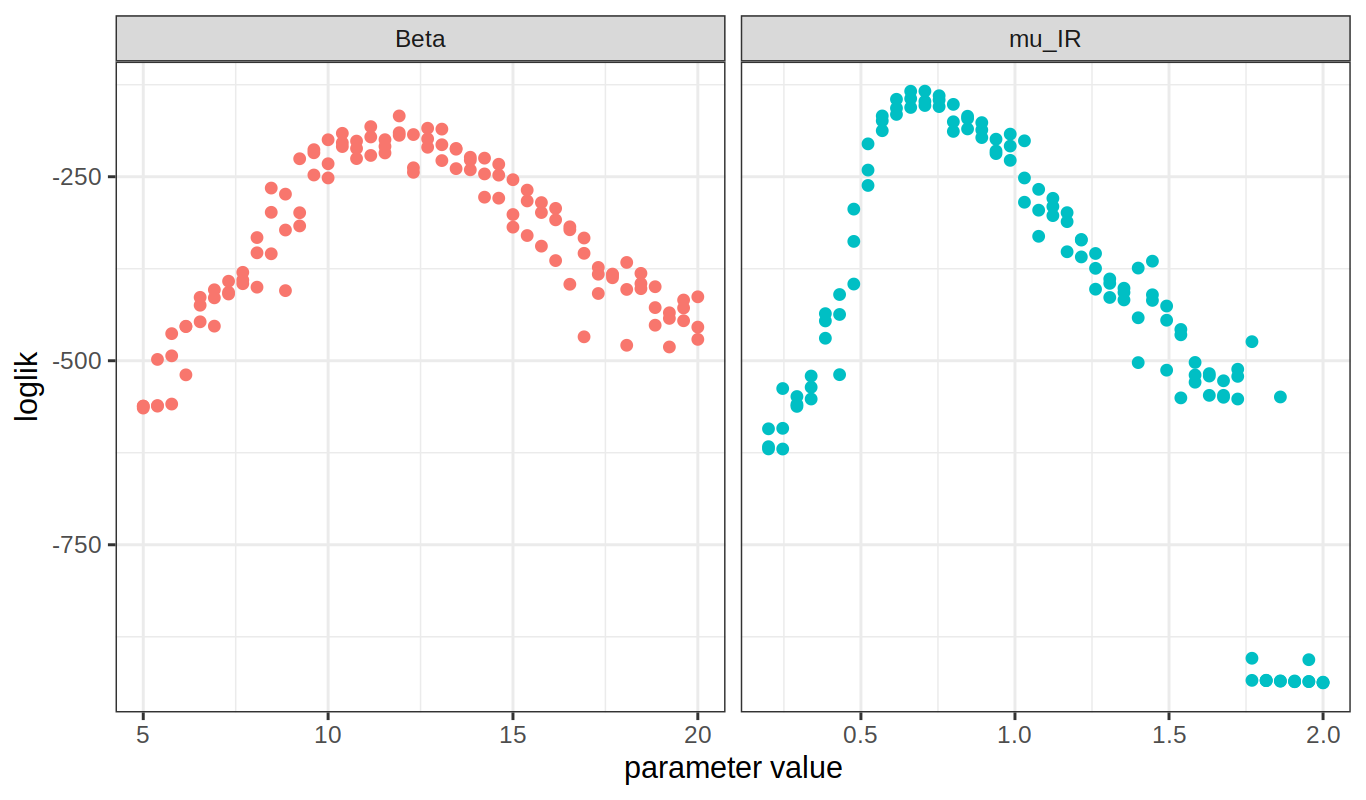• Slices offer a very limited perspective on the geometry of the likelihood surface. When there are only two unknown parameters, we can evaluate the likelihood at a grid of points and visualize the surface directly. expand.grid( Beta=rep(seq(from=10,to=30,length=40),each=3), mu_IR=rep(seq(from=0.4,to=1.5,length=40),each=3), rho=0.5,eta=0.06,N=38000 ) -> p library(foreach) library(doParallel) library(doRNG) registerDoParallel() registerDoRNG(421776444) ## Now we do the computation foreach (theta=iter(p,"row"), .combine=rbind,.inorder=FALSE) %dopar% { library(pomp) measSIR %>% pfilter(params=theta,Np=5000) -> pf theta$loglik <- logLik(pf)
theta
} -> p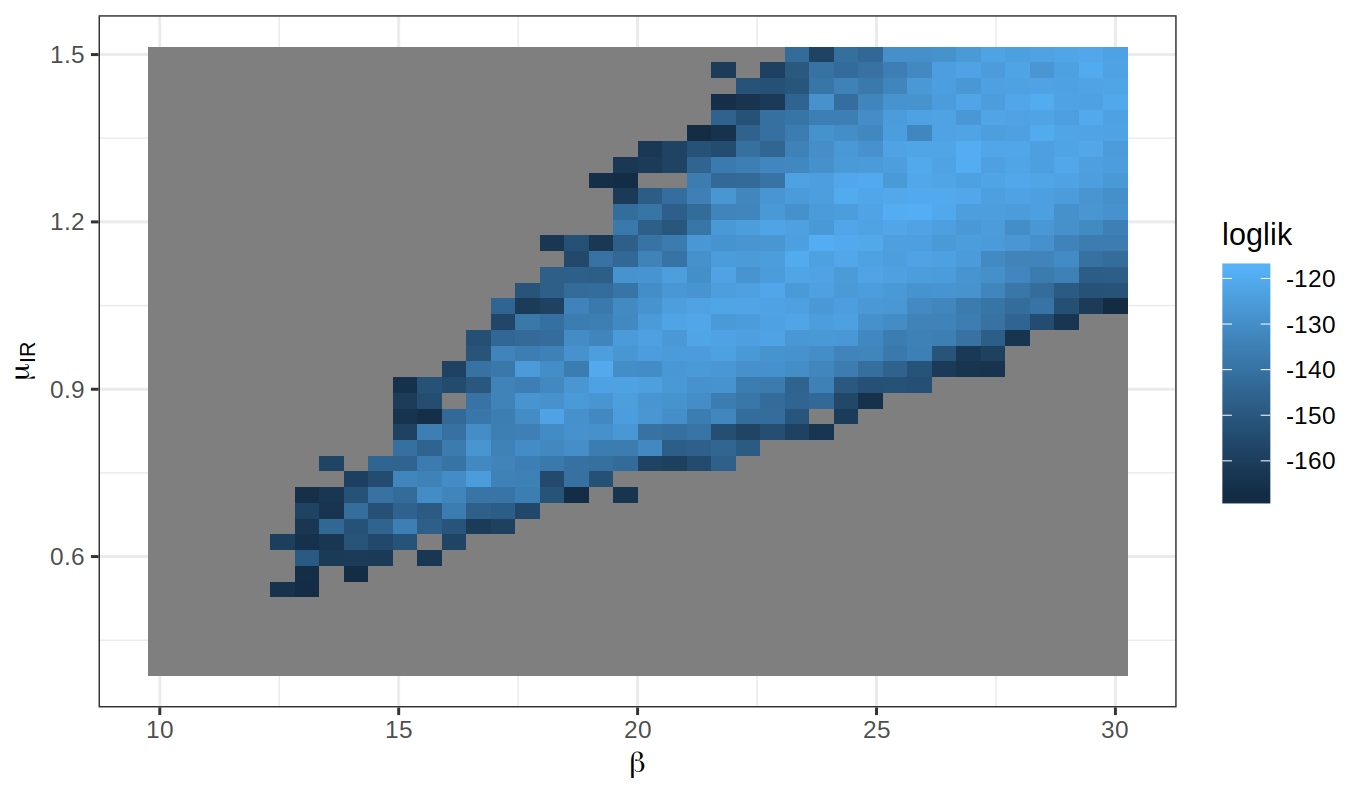In the above, all points with log likelihoods less than 50 units below the maximum are shown in grey.

• Notice some features of the log likelihood surface, and its estimate from the particle filter, that can cause difficulties for numerical methods:
1. The surface is wedge-shaped, so its curvature varies considerably. By contrast, asymptotic theory predicts a parabolic surface that has constant curvature.
2. Monte Carlo noise in the likelihood evaluation makes it hard to pick out exactly where the likelihood is maximized. Nevertheless, the major features of the likelihood surface are evident despite the noise.
• Wedge-shaped relationships between parameters, and nonlinear relationships, are common features of epidemiological dynamic models. We’ll see this in the case studies.

## Exercises

#### Basic Exercise: estimating the expense of a particle-filter calculation

How much computer processing time does a particle filter take, and how does this scale with the number of particles?

First, form a conjecture based upon the description above. Then, test your conjeture by running a sequence of particle filter operations, increasing the number of particles (Np) and measuring the time taken using system.time. Plot your results to test your conjecture.

Worked solution to the Exercise

#### Basic Exercise: log likelihood estimation by particle filtering

Here are some desiderata for a Monte Carlo log likelihood approximation:

• It should have low Monte Carlo bias and variance.

• It should be presented together with estimates of the bias and variance so that we know the extent of Monte Carlo uncertainty in our results.

• It should be computed in a length of time appropriate for the circumstances.

Set up a likelihood evaluation for the flu model, choosing the numbers of particles and replications so that your evaluation takes approximately one minute on your machine.

• Provide a Monte Carlo standard error for your estimate.

• Comment on the bias of your estimate.

• Optionally, use doParallel to take advantage of multiple cores on your computer to improve your estimate.

Worked solution to the Exercise

#### Optional Exercise: one-dimensional likelihood slice

Compute several likelihood slices in the $$\eta$$ direction.

#### Optional Exercise: two-dimensional likelihood slice

Compute a slice of the likelihood in the $$\beta$$-$$\eta$$ plane.

## Maximizing the particle filter likelihood

• Likelihood maximization is key to profile intervals, likelihood ratio tests and AIC as well as the computation of the MLE.

• An initial approach to likelihood maximization might be to stick the particle filter log likelhood estimate into a standard numerical optimizer, such as the Nelder-Mead algorithm.

• In practice this approach is unsatisfactory on all but the smallest POMP models. Standard numerical optimizers are not designed to maximize noisy and computationally expensive Monte Carlo functions.

• Further investigation into this approach is available as a supplement.

• We’ll present an iterated filtering algorithm for maximizing the likelihood in a way that takes advantage of the structure of POMP models and the particle filter.

• First, let’s think a bit about some practical considerations in interpreting the MLE for a POMP.

## More review of likelihood-based inference

### Likelihood-based model selection and model diagnostics

• For nested hypotheses, we can carry out model selection by likelihood ratio tests.

• For non-nested hypotheses, likelihoods can be compared using Akaike’s information criterion (AIC) or related methods.

#### Likelihood ratio tests for nested hypotheses

• The whole parameter space on which the model is defined is $$\Theta\subset\mathbb{R}^D$$.

• Suppose we have two nested hypotheses $\begin{eqnarray} H^{\langle 0\rangle} &:& \theta\in \Theta^{\langle 0\rangle}, \\ H^{\langle 1\rangle} &=& \theta\in \Theta^{\langle 1\rangle}, \end{eqnarray}$ defined via two nested parameter subspaces, $$\Theta^{\langle 0\rangle}\subset \Theta^{\langle 1\rangle}$$, with respective dimensions $$D^{\langle 0\rangle}< D^{\langle 1\rangle}\le D$$.

• We consider the log likelihood maximized over each of the hypotheses, $\begin{eqnarray} \ell^{\langle 0\rangle} &=& \sup_{\theta\in \Theta^{\langle 0\rangle}} \ell(\theta), \\ \ell^{\langle 1\rangle} &=& \sup_{\theta\in \Theta^{\langle 1\rangle}} \ell(\theta). \end{eqnarray}$

• A useful approximation asserts that, under the hypothesis $$H^{\langle 0\rangle}$$, $\ell^{\langle 1\rangle} - \ell^{\langle 0\rangle} \approx (1/2) \chi^2_{D^{\langle 1\rangle}- D^{\langle 0\rangle}},$ where $$\chi^2_d$$ is a chi-squared random variable on $$d$$ degrees of freedom and $$\approx$$ means “is approximately distributed as.”

• We will call this the Wilks approximation.

• The Wilks approximation can be used to construct a hypothesis test of the null hypothesis $$H^{\langle 0\rangle}$$ against the alternative $$H^{\langle 1\rangle}$$.

• This is called a likelihood ratio test since a difference of log likelihoods corresponds to a ratio of likelihoods.

• When the data are IID, $$N\to\infty$$, and the hypotheses satisfy suitable regularity conditions, this approximation can be derived mathematically and is known as Wilks’s theorem.

• The chi-squared approximation to the likelihood ratio statistic may be useful, and can be assessed empirically by a simulation study, even in situations that do not formally satisfy any known theorem.

#### The connection between Wilks’s theorem and profile likelihood

• Suppose we have an MLE, written $$\hat\theta=(\hat\phi,\hat\psi)$$, and a profile log likelihood for $$\phi$$, given by $$\ell_\mathrm{profile}(\phi)$$.

• Consider the likelihood ratio test for the nested hypotheses $\begin{eqnarray} H^{\langle 0\rangle} &:& \phi = \phi_0, \\ H^{\langle 1\rangle} &:& \mbox{\phi unconstrained}. \end{eqnarray}$

• We can check what the 95% cutoff is for a chi-squared distribution with one degree of freedom,

qchisq(0.95,df=1)
##  3.841459
• Wilks’s theorem then gives us a hypothesis test with approximate size $$5\%$$ that rejects $$H^{\langle 0\rangle}$$ if $$\ell_\mathrm{profile}(\hat\phi)-\ell_\mathrm{profile}(\phi_0)<3.84/2$$.

• It follows that, with probability $$95\%$$, the true value of $$\phi$$ falls in the set $\big\{\phi: \ell_\mathrm{profile}(\hat\phi)-\ell_\mathrm{profile}(\phi)<1.92\big\}.$ So, we have constructed a profile likelihood confidence interval, consisting of the set of points on the profile likelihood within 1.92 log units of the maximum.

• This is an example of a general duality between confidence intervals and hypothesis tests.

#### Akaike’s information criterion (AIC)

• Likelihood ratio tests provide an approach to model selection for nested hypotheses, but what do we do when models are not nested?

• A more general approach is to compare likelihoods of different models by penalizing the likelihood of each model by a measure of its complexity.

• Akaike’s information criterion AIC is given by $\textrm{AIC} = -2\,\ell(\hat{\theta}) + 2\,D$ “Minus twice the maximized log likelihood plus twice the number of parameters.”

• We are invited to select the model with the lowest AIC score.

• AIC was derived as an approach to minimizing prediction error. Increasing the number of parameters leads to additional overfitting which can decrease predictive skill of the fitted model.

• Viewed as a hypothesis test, AIC may have weak statistical properties. It can be a mistake to interpret AIC by making a claim that the favored model has been shown to provides a superior explanation of the data. However, viewed as a way to select a model with reasonable predictive skill from a range of possibilities, it is often useful.

• AIC does not penalize model complexity beyond the consequence of reduced predictive skill due to overfitting. One can penalize complexity by incorporating a more severe penalty that the $$2D$$ term above, such as via BIC.

• A practical approach is to use AIC, while taking care to view it as a procedure to select a reasonable predictive model and not as a formal hypothesis test.